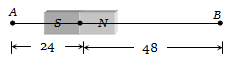You’ve reached the end of your free Videos limit.
#11 | Apparent & True Dip
(Physics) > Magnetism and Matter
Unable to watch the video, please try another server
Related Practice Questions :

1.

2.

3.

4.  ${\mathrm{tan}}^{-1}\left(\frac{2}{\sqrt{3}}\right)$

High Yielding Test Series + Question Bank - NEET 2020

Difficulty Level:

The vertical component of the earths magnetic field is zero at

(1) Magnetic poles

(2) Magnetic equator

(3) Geographic poles

(4) Geographic equator

High Yielding Test Series + Question Bank - NEET 2020

Difficulty Level:

A neutral point is obtained at the centre of a vertical circular coil carrying current. The angle between the plane of the coil and the magnetic meridian is :

a) 0                           (b) 45°

(c) 60°                       (d) 90°

High Yielding Test Series + Question Bank - NEET 2020

Difficulty Level:

A bar magnet of length 3 cm has points A and B along its axis at distances of 24 cm and 48 cm on the opposite sides. Ratio of magnetic fields at these points will be(a) 8                               (b)$\frac{1}{2\sqrt{2}}$

(c) 3                               (d) 4

High Yielding Test Series + Question Bank - NEET 2020

Difficulty Level:

If the angles of dip at two places are 30o and 45o respectively, then the ratio of horizontal  components of earth's magnetic field at the two places will be

(a) $\sqrt{3}:\sqrt{2}$                       (b) $1:\sqrt{2}$

(c) $1:\sqrt{3}$                          (d) 1:2